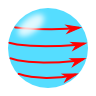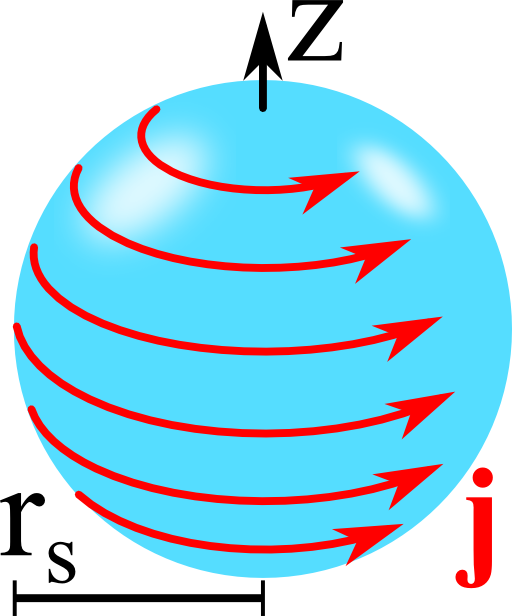## A Magnetic Sphere with Surface CurrentUsing the magnetostatic potential can be extremely useful to calculate magnetostatic problems. However, it can only be defined if no currents are present. In this problem you will discover how we can still use the potential in situations of strongly confined surface currents.

## Problem StatementConsider a sphere with relative permeability $$\mu_{r}$$ and radius $$r_{s}$$. On the surface of the sphere there shall be a current flowing in azimuthal $$\varphi$$-direction, strongest at the equator: $$\mathbf{j}\left(\mathbf{r}\right)=j_{0}\delta\left( r-r_{s} \right)\sin\theta\mathbf{e}_{\varphi}$$. Calculate the magnetic induction $$\mathbf{B}\left(\mathbf{r}\right)$$ everywhere. Discuss what further steps you would have to take if the surface current obeys a more complicated form.

## Background: Artificial Magnetic Materials

In the optical frequency range, magnetic light-matter-interactions are usually very weak. This means that relative permeabilities $$\mu_r$$ are very close to unity. Nevertheless, strong magnetic interactions would have a lot of incredible applications like perfect lenses or cloaking devices. The reason is that such devices rely on a spatially varying permittivity $$\epsilon\left(\mathbf{r},\omega\right)$$ and permeability $$\mu\left(\mathbf{r},\omega\right)$$. Hence, there is a lot of interest to design artificial materials that have a somewhat stronger magnetic interaction than ordinary matter.

One way to get such an artificial magnetic material is described in the publication "Self-Assembled Plasmonic Core–Shell Clusters with an Isotropic Magnetic Dipole Response in the Visible Range" by Mühlig et al., ACS Nano 5 (2011). There, the authors attach a lot of small metallic nanospheres with radii around 10 nm onto a larger dielectric sphere with a radius of around 100 nm. The metallic spheres then act as an effective medium that can have an extremely high permittivity at certain frequencies. Then, an excitation field causes the electrons in the metal to oscillate around the magnetic field vector. This (oscillating!) ring current leads to an effective coupling to the magnetic field. In a certain sense, we ask in our problem for the inverse situation: What kind of magnetic field is caused by a certain azimuthal surface current?